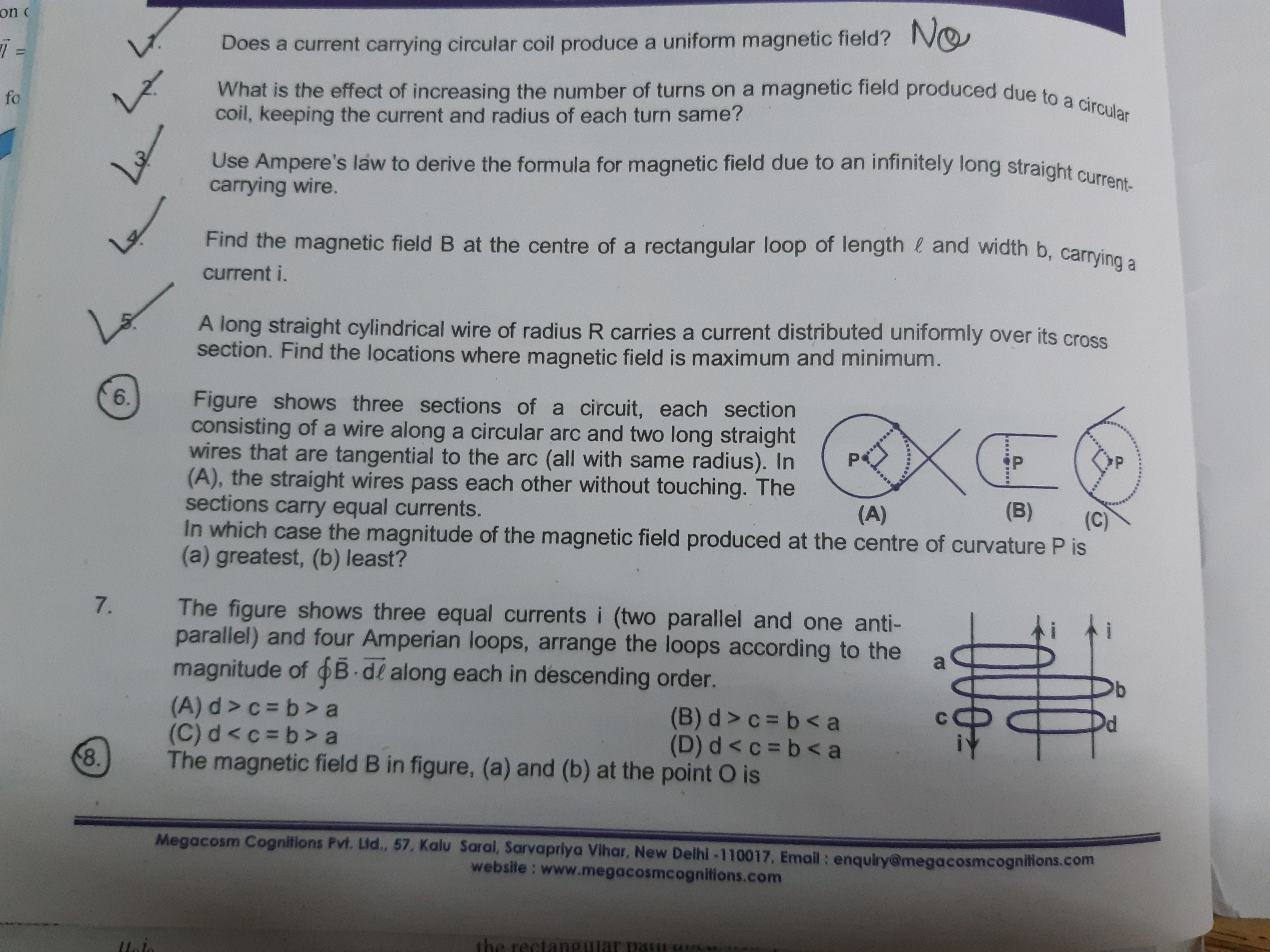# Doubt from magnetism

Consider an infinitely long cylinderical ampere loop with radius d
\displaystyle \int B dl= B×2πd=\mu_0 I_{en}
Here d is the distance from the wire

1 Like

Bro can you please tell how to write myu nought in math jax ?

\mu_0 Write this in$......$

1 Like

Question 6 and 8th in 8th first figure explain i am not able to understandIn 6 you have to find the effective magnetic field
At P due to circular arc and Two straight lines

In 8 you should find current in every branch due to resistance

(\vec{A} × \vec{B})= \vec{C}
Then C will be perpendicular to both A and B
A may be perpendicular to B or may not be

1 Like

R=\frac{mv}{qB}
Manipulate this equation to get correct option
When their momentum is same then their velocity will not be same

1 Like

Question 1 For B part force and direction will be ?

\vec{F} = i\vec{L}× \vec{B}
\vec{L} is along the current

1 Like

In B the force will be outward from the plane of paper

1 Like

Ignore post 14 i have solved that i am confused in question 4 one teacher telling option A is correct but answer key says option C is correct please tell which is correct along with working.

Is magnetic moment independent of magnetic field ?

Magnetic moment \vec{M} =Ni\vec{A}

2sigma x π x R/3m for 13th?

It is given 2 pi sigmanot R^2/5m for 13th